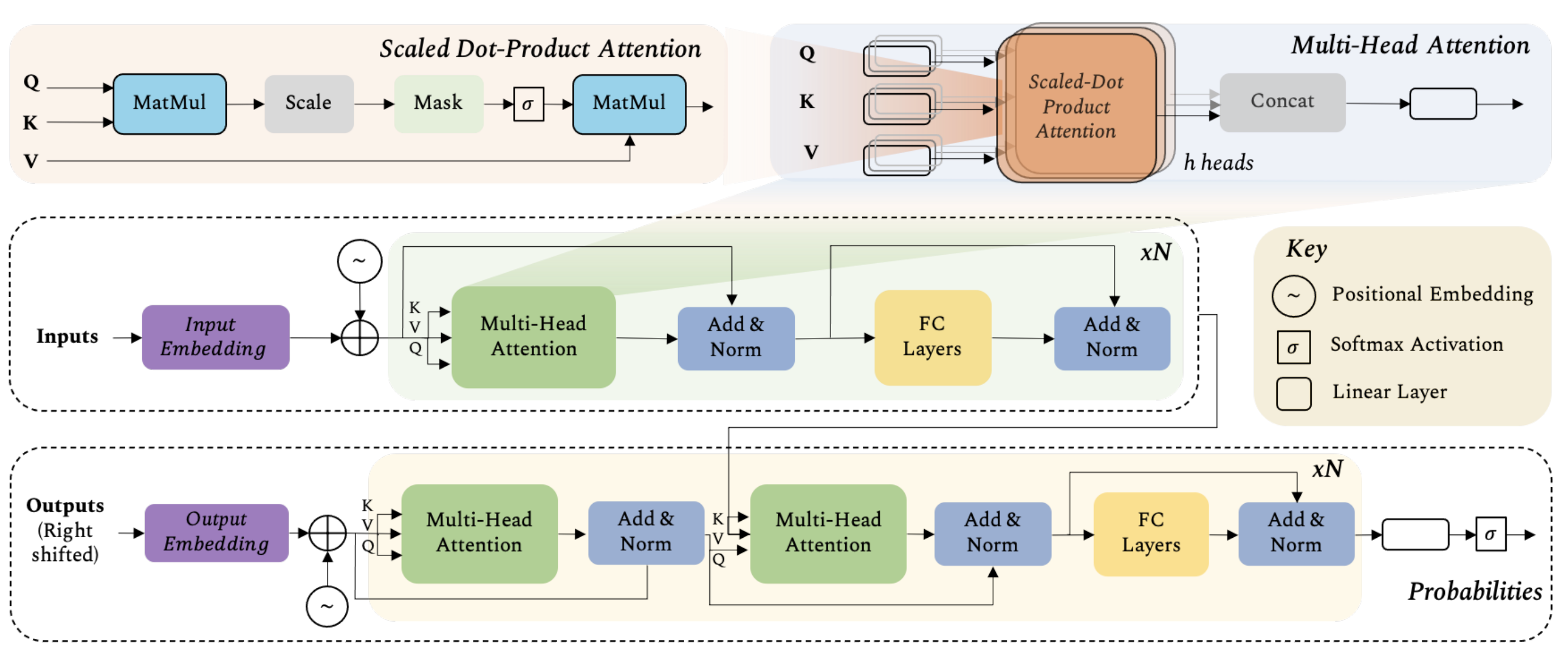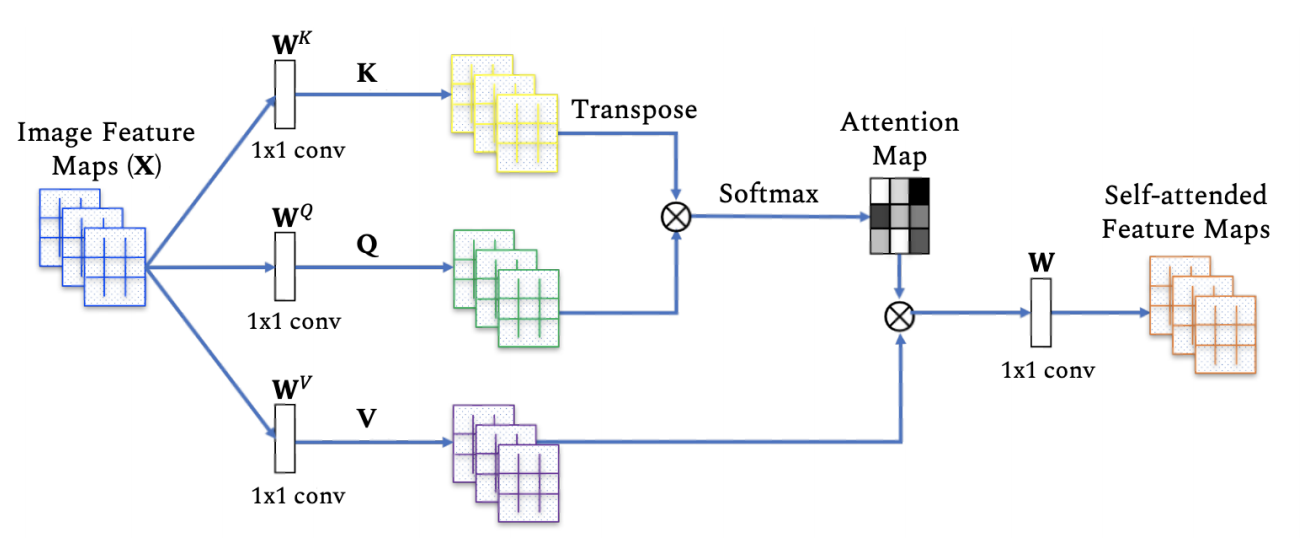# Transformer Introduction

This is my reading note for Transformers in Vision: A Survey. Transformers enable modeling long dependencies between input sequence elements and support parallel processing of sequence as compared to recurrent networks e.g., Long short-term memory (LSTM). Different from convolutional networks, Transformers require minimal inductive biases for their design and are naturally suited as set-functions. Furthermore, the straightforward design of Transformers allows processing multiple modalities (e.g., images, videos, text and speech) using similar processing blocks and demonstrates excellent scalability to very large capacity networks and huge datasets.

There exist two key ideas that have contributed towards the development of transformer models.

• The first one is self attention, which allows capturing ‘long-term’ information and dependencies between sequence elements as compared to conventional recurrent models that find it challenging to encode such relationships.
• The second key idea is that of pre-training on a large (un)labelled corpus in a (un)supervised manner, and subsequently fine-tuning to the target task with a small labeled dataset.# Self Attention

Transformer is based on self attention. The self attention block could be shown as below:Here image feature maps $$X\in\mathbb{R}^{n\times d}$$, with three learnable weight matrics to transform keys $$W^K\in\mathbb{R}^{n\times d_K}$$, query $$W^Q\in\mathbb{R}^{n\times d_Q}$$ and value $$W^V\in\mathbb{R}^{n\times d_V}$$ (typically $$d_K=d_Q=d_V$$), then output $$Z\in\mathbb{R}^{n\times d_v}$$ can be computed as:

$Z=softmax(\frac{QK^T}{\sqrt(d_q)}V)$

For a given entity in the sequence, the self-attention basically computes the dot-product of the query with all keys, which is then normalized using softmax operator to get the attention scores. Each entity then becomes the weighted sum of all entities in the sequence, where weights are given by the attention scores.

In order to encapsulate multiple complex relationships amongst different elements in the sequence, the multi-head attention comprises multiple selfattention blocks (h = 8 in the original Transformer model). Each block has its own set of learnable weight matrices $$\{W^{Q_i},W^{V_i},W^{K_i}\}\text{ for }i=0,1,h-1$$. For an input X, the output of the h self-attention blocks in multihead attention is then concatenated into a single matrix $$[Z_0,Z_1,...,Z_{h-1}]\in\mathbb{R}^{n\times hd_v}$$ and projected onto a weight matrix $$W\in\mathbb{R}^{hd_v\times d}$$.

# Transformer

Now we could discuss the transformer. It has an encoder-decoder structure. The encoder (middle row) consists of six identical blocks (i.e., N=6 in the figure in the top), with each block having two sub-layers: a multi-head self-attention network, and a simple positionwise fully connected feed-forward network. Residual connections alongside layer normalization are employed after each block as in the figure in the top. Self-attention layer only performs aggregation while the feed-forward layer performs transformation.

Similar to the encoder, the decoder (bottom row) in the Transformer model comprises six identical blocks. Each decoder block has three sub-layers, first two (multi-head self-attention, and feedforward) are similar to the encoder, while the third sublayer performs multi-head attention on the outputs of the corresponding encoder block.

The decoder takes inputs from the encoder as well as the previous outputs to predict the next word of the sentence in the translated language.

## Positional Encoding

Positional encodings are added to the input sequence to capture the relative position of each word in the sequence. Positional encodings have the same dimensions as the input $$d=512$$, and can be learned or pre-defined e.g., by sine or cosine functions.

# Pre-Training

Self-attention based Transformer models generally operate in a two-stage training mechanism. First, pre-training is performed on a large-scale dataset (and sometimes a combination of several available datasets) in either a supervised or an unsupervised manner. Later, the pre-trained weights are adapted to the downstream tasks using small-mid scale datasets.

As nicely summarized by Y. LeCun, the basic idea of SSL is to fill in the blanks, i.e., try to predict the occluded data in images, future or past frames in temporal video sequences or predict a pretext task e.g., the amount of rotation applied to inputs, the permutation applied to image patches or the color of a gray-scale image.

Written on April 14, 2021